A solution containing 8.6 g urea in one litre was found to be isotonic with a 5% (wt./vol.) solution of an organic non-volatile solute. The molecular weight of latter is:

Anonymous User Chemistry Solutions 13 Mar, 2020 88 views

Dry air was passed successively through a solution of 5 g of a solute in 180 g of water and then through pure water. The loss in weight of solution was 2.50 g and that of pure solvent 0.04 g. The molecular weight of the solute is:

Anonymous User Chemistry Solutions 13 Mar, 2020 72 views

20g of sample of Ba(OH)2 is dissolved in 50 mL of 0.1 N HCl solution. The excess of HCl was titrated with 0.1 N NaOH. The volume of NaOH used was 20 ml. The percentage of Ba(OH)2 in the sample is

Anonymous User Chemistry stoichiometry 12 Mar, 2020 115 views

A metal oxide has the formula Z2O3. It can be reduced by hydrogen to give free metal and water. 0.1596g of the metal oxide requires 6 mg of hydrogen for complete reduction. The atomic weight of the metal is

Anonymous User Chemistry stoichiometry 12 Mar, 2020 192 views

20 ml of a H3PO4 solution needs 40 ml of 0.1 M NaOH to convert it into sodium dihydrogen phosphate. How much volume of 0.1 M Ca(OH)2 is needed to neutralise the same volume of same H3PO4 completely

Anonymous User Chemistry stoichiometry 12 Mar, 2020 644 views

## The equivalent weight of iron in  would beThe equivalent weight of iron in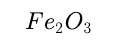would be

Anonymous User Chemistry stoichiometry 12 Mar, 2020 62 views

The vapour density of undecomposed N2O4 is 46. When heated, vapour density decreases to 24.5 due to its dissociation to NO2. The % dissociation of N2O4 at the final temperature is

Anonymous User Chemistry Equilibrium 12 Mar, 2020 538 views

## For the reaction  the forward reaction at constant  temperatured is favoured byFor the reaction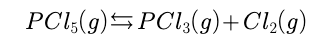the forward reaction at constant  temperatured is favoured by

Anonymous User Chemistry Equilibrium 12 Mar, 2020 56 views

In the system, LaCl3(s) + H2O (g)+heat  ------------>.   LaClO(s)+2HCl (g), equilibrium is established. More water vapour is added to reestablish the equilibrium. The pressure of water vapour is doubled. The factor by which pressure of HCl is changed is

Anonymous User Chemistry Equilibrium 12 Mar, 2020 351 views

For a reaction A(g) + B(g) ---------> C(g) + D(g), the initial concentration of A and B are equals but the equilibrium concentration of C is twice that of equilibrium concentration of A. Then K is

Anonymous User Chemistry Equilibrium 12 Mar, 2020 58 views

The partial pressure of CH3OH (g), CO(g) and H2(g) in equilibrium mixture for the reaction

CO(g) + 2H2(g) ----> CH3OH(g)

are 2.0, 1.0, and 0.1 atm respectively at 427°C. The value of Kp for decomposition of CH3OH to CO and H2 is

Anonymous User Chemistry Equilibrium 12 Mar, 2020 298 views

The equilibrium constant for the reaction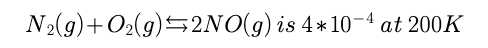In the presence of a catalyst the equilibrium is attained 10 times faster. Therefore the equilibirum constant in presence of the catalyst at 200 K is

Anonymous User Chemistry Equilibrium 12 Mar, 2020 57 views

In a reaction at equilibrium ‘X’ moles of the reactant A decomposes to give 1 mole each of C and D. If the fraction of A decomposed at equilibrium is independent of initial concentration of A then the value ‘X’ is

Anonymous User Chemistry Equilibrium 12 Mar, 2020 89 views

Calculate Kc for the reaction A(g) + 2B(g) 3C(g) when the reaction was started with 2 moles/litre of A and 3 moles/litre of B. The equilibrium concentration of C is 3 mole/litre.

Anonymous User Chemistry Equilibrium 09 Mar, 2020 64 views

## In the equation  the dimensional formula of w is :In the equation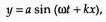the dimensional formula of w is :

Anonymous User Physics Units and Dimension 07 Mar, 2020 60 views

The equation of alternating current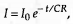where t is time, C is capacitance and R is resistance of coil, then the dimensions of CR is:

Anonymous User Physics Units and Dimension 07 Mar, 2020 64 views

A particle moves with simple harmonic motion in a straight line. In first τ s, after starting from rest it

travels a distance a, and in next τ s it travels 2a, in same direction, then :

(1) amplitude of motion is 4a

(2) time period of oscillations is 6τ

(3) amplitude of motion is 3a

(4) time period of oscillations is 8τ

Anonymous User Physics 07 Mar, 2020 65 views